# Machine Learning Series: NYC Yellow Taxi Tips PredictionMay 17, 2022Pamphile Roy

Bodo allows machine learning practitioners to rapidly explore data and build complex pipelines. Using Bodo, developers can seamlessly scale their codes from using their own laptop to using Bodo's platform. In this series, will present a few hands-on.

The following example is based on the 2019 NYC Yellow Cab trip record data made available by the NYC Taxi and Limousine Commission (TLC). This dataset has been widely used on Kaggle and elsewhere. Todd Schneider has written a nice in-depth analysis of the dataset.

After exploring the data, we will use a regression model to predict taxi tips.

A notebook version of this blog post is available here.

### Prelude

#### Bodo

In this post, we will run the code using Bodo. It means that the data will be distributed in chunks across processes. Bodo's documentation provides more information about the parallel execution model.

If you want to run the example using Pandas only (without Bodo), simply comment out lines with the Bodo JIT decorator (@bodo.jit) from all the code cells. Bodo is as easy as that.

Note: Start an IPyParallel cluster (skip if running on Bodo's Platform)

#### Import all packages

These are the main packages we are going to work with:

• Bodo to parallelize Python code automatically;
• IPyParallel for setting up a local cluster (if not on Bodo's platform);
• Pandas to manipulate tabular data structures;
• Seaborn and Matplotlib for data visualization; and
• Scikit-Learn to build and evaluate regression models.

We use the data from the year 2019 . We can fetch the data directly from the NYC Taxi & Limousine Commission; alternatively, we can get it from one of Bodo's AWS S3 open buckets no AWS credentials required).

In the following, we load the dataset directly from AWS using the URI s3://bodo-examples-data/nyc-taxi/yellow_tripdata_2019.csv.

Note that the data is in fact distributed on all cores you allocated for Bodo.

By default, the data is distributed to all available engines; that is, the rows of the DataFrame taxi are split into four partitions and sent to each of the cores. This allows embarrassingly parallel algorithms—like computing sums—to scale with the data and resources at hand.

### Exploratory Analysis

The dataset consist of 18 columns; see the TLS documentation for more details on the meanings of each field. Our goal here is to predict the tip amount of a ride using some features.

Before doing anything, it might be interesting for us to look at the raw data to see if we can extract patterns.

There are a few preprocessing steps we can make based on insights available from examining the dataset. The data sheet states "cash tips are not included," so we'll look at credit card transactions exclusively. Other fields are unclear and show rows have some suspicious entries (e.g., a trip of length 0 miles with no charge but a tip of \$50). To mitigate the presence of outliers and data pollution, we modify the data beforehand by:

1. removing records with a price of \$0.00;
2. removing records with a trip distance of < 0.01 miles;
3. keeping only records with credit card transactions;
4. computing tip_fraction only for these relevant records; and
5. processing the date into discrete values (day of the week, day of the month, etc.).

First, let's have a look at both tip_fraction and fare_amount distributions. The dataset is randomly sub-sampled to facilitate computations outside Bodo.

The following snippet mixes Bodo with normal Pandas and Seaborn/Matplotlib code. This is handy when a particular feature or package is not yet supported by Bodo. Notice we use the smaller DataFrame sample to make plotting easier.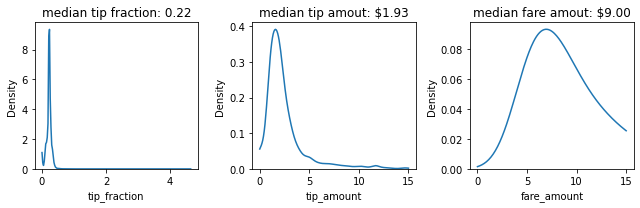From left to right. Probability density functions for the tip fraction, tip  amount and fare amount.

We can use the whole dataset with Bodo to compute descriptive statistics.

Even after filtering the data, there are still interesting things to note on the tip fraction. Apparently someone tipped 4500x the fare...

We often use correlation matrices in machine learning to identify important features. And, in this case, we want to know which features can be used to predict tips.

The correlation matrix by itself is large and since only the tip amount is of interest, there is no need to plot everything.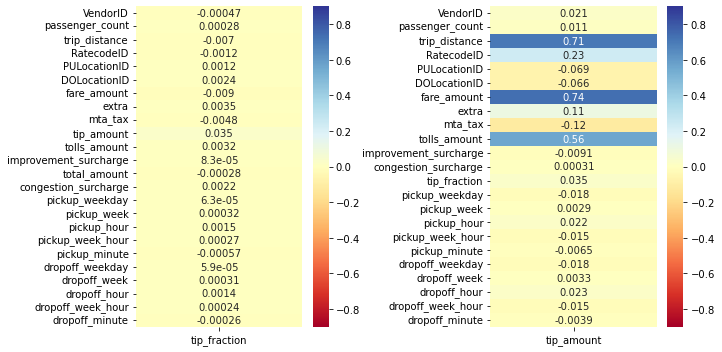Left: correlations between tip fraction and other parameters. Right: correlations between tip amount and other parameters

These correlation plots suggest that the tip_fraction is mostly uncorrelated from all the other parameters. The (absolute) tip_amount itself is strongly correlated to the tolls_amount, trip_distance and fare_amount.

### Modeling Tip Amount

Based on the preceding correlation plots, we prepare the dataset by only selecting the tolls_amount, trip_distance and fare_amount as the exogenous variables in our regression model. We extract this new dataset from the filtered taxi dataset using typical column selection. We then split the DataFrame into a training and a testing set with a ratio of 70%/30%, respectively–which is standard practice in machine learning.

Next, we train a model and use the test data to evaluate the RMSE (Root-Mean-Square-Error) and the R^2R2 statistic (computed with the functions mean_squared_error & r2_score respectively, both from Scikit-Learn's metrics submodule).

The RMSE and R^2R2 scores give us a decent first idea of the performance of the model. We can also analyze the density of the output.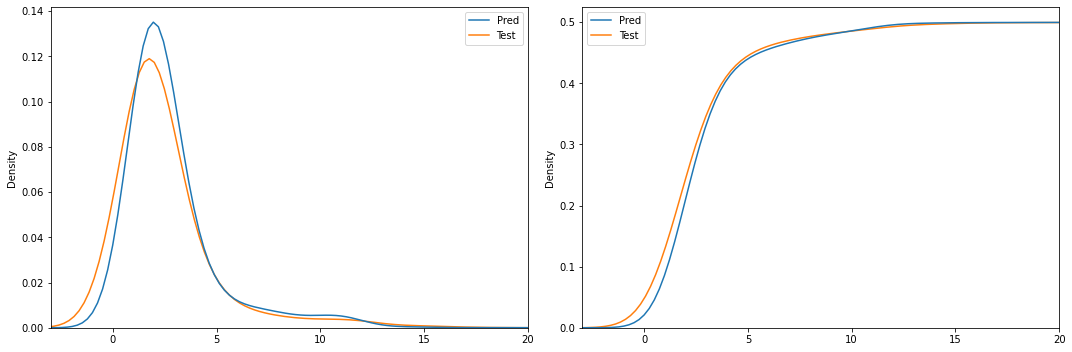Comparison between observed and predicted probability (left)/cumulative  (right) density functions of the tip fraction

The model is of modest quality but can be useful to provide first estimate.

This concludes this example. Bodo helped us to control and efficiently use the computational resources of our machine. With very little adjustment, we were able to speed up and scale our analysis. Bodo provides an extensive API and supports many well known packages to accommodate for any kind of machine learning pipelines.

Note: Don't forget to stop your cluster: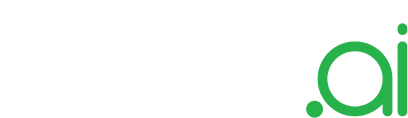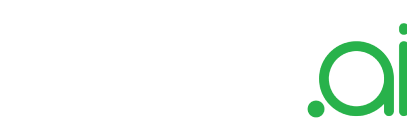PRODUCT
TECHNOLOGY